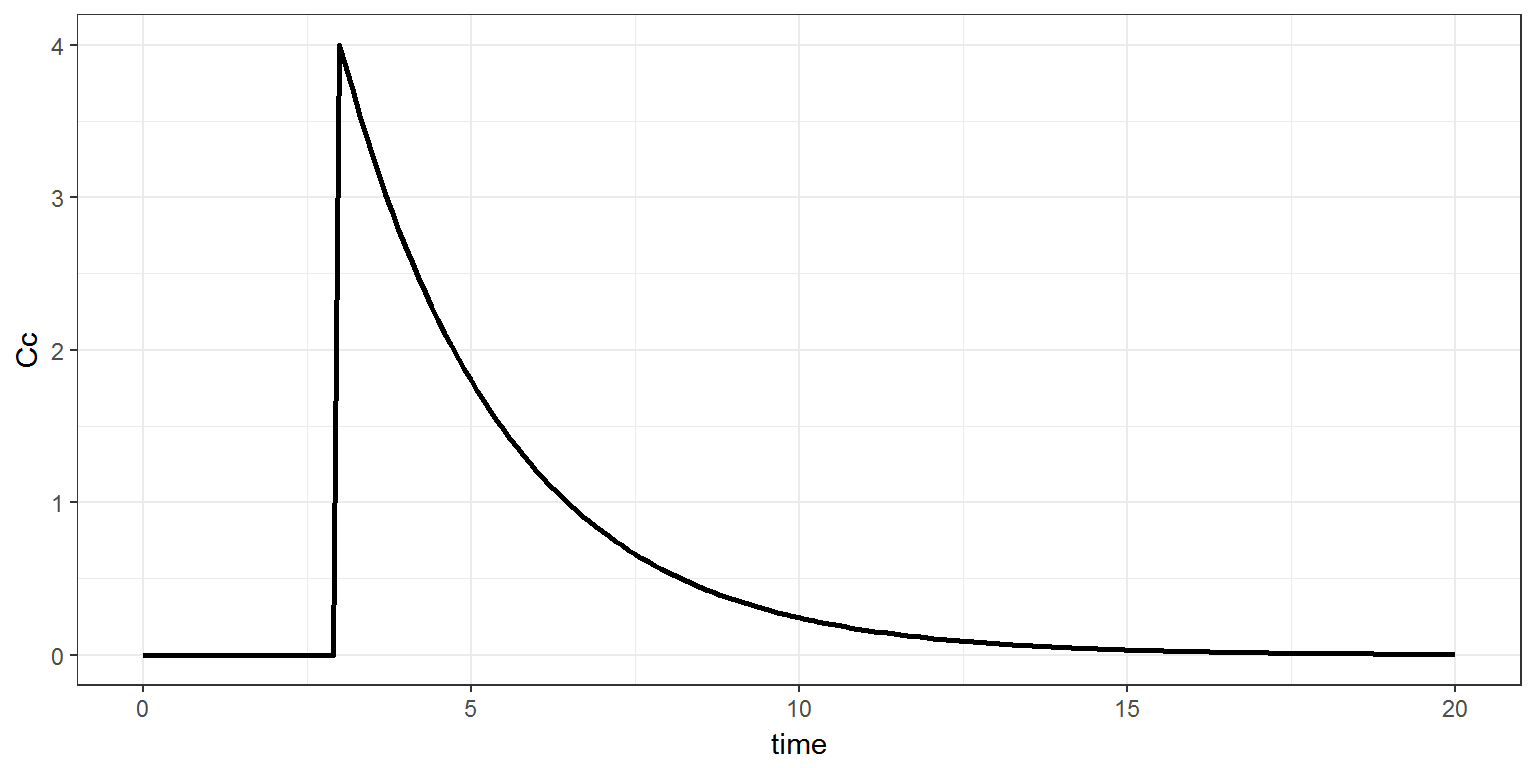R script: pk1.R

Mlxtran code: model/pk1a.txt ; model/pk1b.txt

$$\newcommand{\esp}{\mathbb{E}\left(#1\right)} \newcommand{\var}{\mbox{Var}\left(#1\right)} \newcommand{\deriv}{\dot{#1}(t)} \newcommand{\prob}{ \mathbb{P}\!(#1)} \newcommand{\eqdef}{\mathop{=}\limits^{\mathrm{def}}} \newcommand{\by}{\boldsymbol{y}} \newcommand{\bc}{\boldsymbol{c}} \newcommand{\bpsi}{\boldsymbol{\psi}} \def\pmacro{\texttt{p}} \def\like{{\cal L}} \def\llike{{\cal LL}} \def\logit{{\rm logit}} \def\probit{{\rm probit}} \def\one{{\rm 1\!I}} \def\iid{\mathop{\sim}_{\rm i.i.d.}} \def\simh0{\mathop{\sim}_{H_0}} \def\df{\texttt{df}} \def\res{e} \def\xomega{x} \newcommand{\argmin}{{\rm arg}\min_{#1}} \newcommand{\argmax}{{\rm arg}\max_{#1}} \newcommand{\Rset}{\mbox{\mathbb{R}}} \def\param{\theta} \def\setparam{\Theta} \def\xnew{x_{\rm new}} \def\fnew{f_{\rm new}} \def\ynew{y_{\rm new}} \def\nnew{n_{\rm new}} \def\enew{e_{\rm new}} \def\Xnew{X_{\rm new}} \def\hfnew{\widehat{\fnew}} \def\degree{m} \def\nbeta{d} \newcommand{\limite}{\mathop{\longrightarrow}\limits_{#1}} \def\ka{k{a}} \def\ska{k{\scriptscriptstyle a}} \def\kel{k{e}} \def\skel{k{\scriptscriptstyle e}} \def\cl{C{\small l}} \def\Tlag{T\hspace{-0.1em}{lag}} \def\sTlag{T\hspace{-0.07em}{\scriptscriptstyle lag}} \def\Tk{T\hspace{-0.1em}{k0}} \def\sTk{T\hspace{-0.07em}{\scriptscriptstyle k0}} \def\thalf{t{1/2}} \newcommand{\Dphi}{\partial_\pphi #1} \def\asigma{a} \def\pphi{\psi} \newcommand{\stheta}{{\theta^\star}} \newcommand{\htheta}{{\widehat{\theta}}}$$

# 1 Introduction

Once a drug is administered, we usually describe subsequent processes within the organism by the pharmacokinetics (PK) process known as ADME: absorption, distribution, metabolism, excretion.

A PK model is a dynamical system mathematically represented by a system of ordinary differential equations (ODEs) which describe transfers between compartments and elimination from the central compartment.

See this web animation for more details.

# 2 Example

## 2.1 The PK model

Let us start with a very basic one compartment model for intraveinous (iv) administration with linear elimination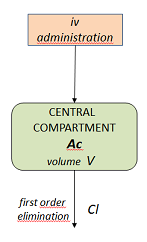\begin{align} \deriv{A_c} &= - k \, A_c(t) \\ A_c(t) &= 0 \quad \text{for} \ \ t<0 \end{align} Here, $$A_c(t)$$ and $$C_c(t)=A_c(t)/V$$ are, respectively, the amount and the concentration of drug in the central compartment at time $$t$$.

Doses can then arrive in the central compartment at any times $$\tau_1$$, $$\tau_2, \ldots$$. An iv bolus administration assumes that $A_c(\tau_j^+) = A_c(\tau_j^-) + D_j$ where $$D_j$$ is the amount of drug administrated at time $$\tau_j$$.

On the other hand, an iv infusion with rate $$R_j$$ assumes that $A_c(t) = A_c(\tau_j) + \min(R_j\, (t-\tau_j) , D_j),$ for $$\tau_j \leq t \leq \tau_{j+1}$$

## 2.2 The Mlxtran model file

This model is implemented in the file pk1a.txt using the pkmodel function, which recognizes the model according to the list of its input arguments.

[LONGITUDINAL]
input = {V, Cl}

EQUATION:
Cc = pkmodel(V, Cl)


Remark: The pkmodel function is extremely convenient for implementing easily about 150 basic PK models. Nevertheless, there exists several other ways to implement the same PK model with Mlxtran.

It is possible, for instance, to use some PK macros defined in the block PK:

[LONGITUDINAL]
input = {V, Cl}

PK:
compartment(cmt=1, amount=Ac, volume=V)
iv(cmt=1)
elimination(cmt=1, Cl)
Cc = Ac/V


It is also possible to implement the PK model as a system of ODEs in the block EQUATION and define the target compartment (here the central compartment) in the block PK.

[LONGITUDINAL]
input = {V, Cl}

PK:
depot(target=Ac)

EQUATION:
k = Cl/V
ddt_Ac = -k*Ac
Cc=Ac/V


The pkmodelfunction and the PK macros should be preferred when it is possible since they use an analytic solution when the system is linear instead of soving an ODE system.

Let us now use this model with simulx for computing the concentration in the central compartment between time 0 and 20, when a dose of 40g is administered at time 40 as an iv bolus. We will assume the following PK parameters in the next examples: $V=10\,\text{l} \quad ; \quad Cl=4\, \text{l/h}$

The dosage regimen is defined in the input argument treatment of simulx. For an iv bolus administration, treatment has two fields: time, the time(s) of administration, and amount, the amount(s) of drug.

adm <- list(time=3, amount=40)

Cc  <- list(name='Cc',time=seq(from=0, to=20, by=0.1))
p   <- c(V=10, Cl=4)

res <- simulx(model='model/pk1a.txt',
parameter=p,
output=Cc,

print(ggplot(data=res$Cc, aes(x=time, y=Cc)) + geom_line(size=1))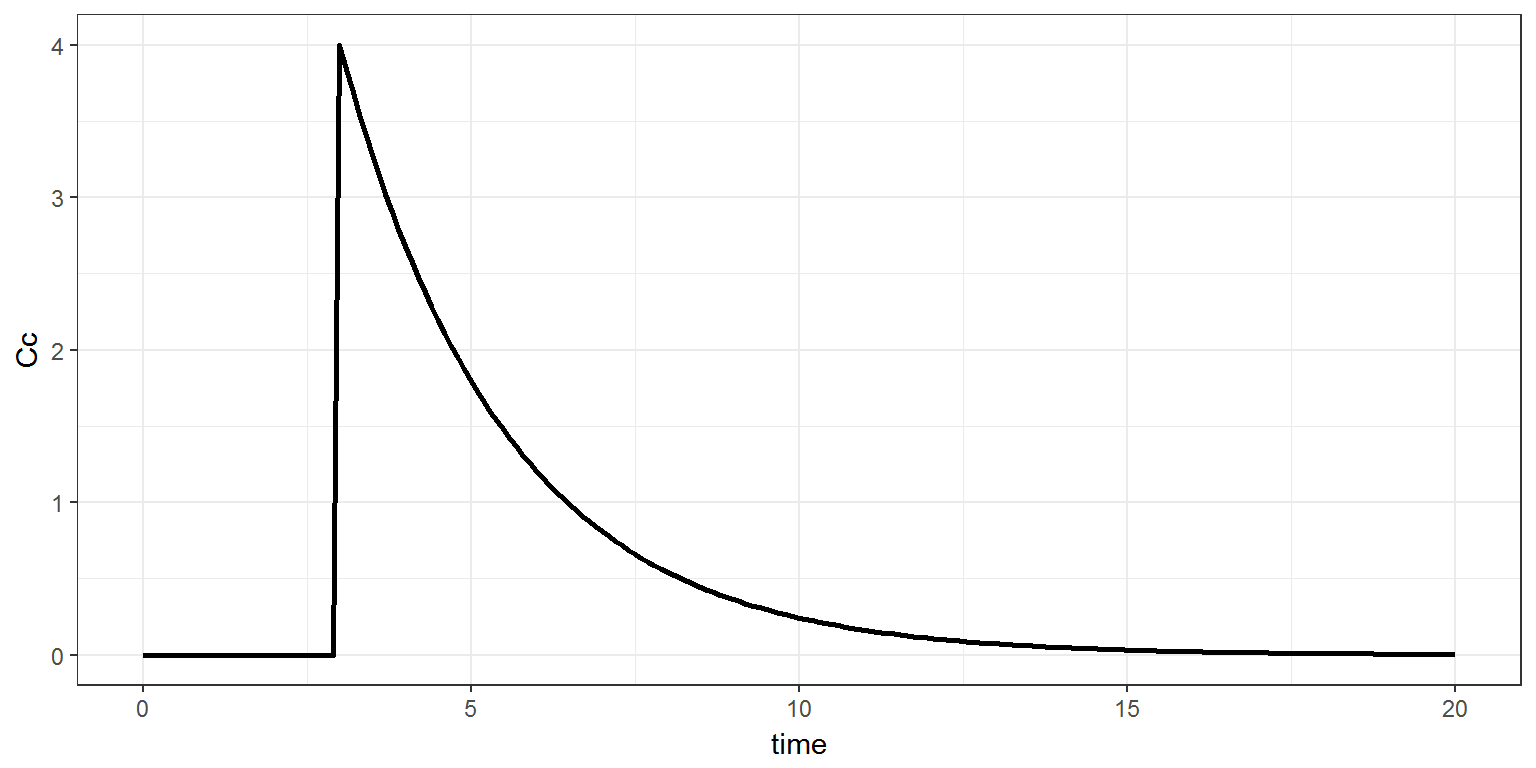## 2.4 Multiple doses administration Multiple doses of a same amount are obtained by defining adm$time as a vector

adm <- list(time=c(1, 7, 13), amount=40)
res <- simulx(model='model/pk1a.txt', parameter=p, output=Cc, treatment=adm)
print(ggplot(data=res$Cc, aes(x=time, y=Cc)) + geom_line(size=1))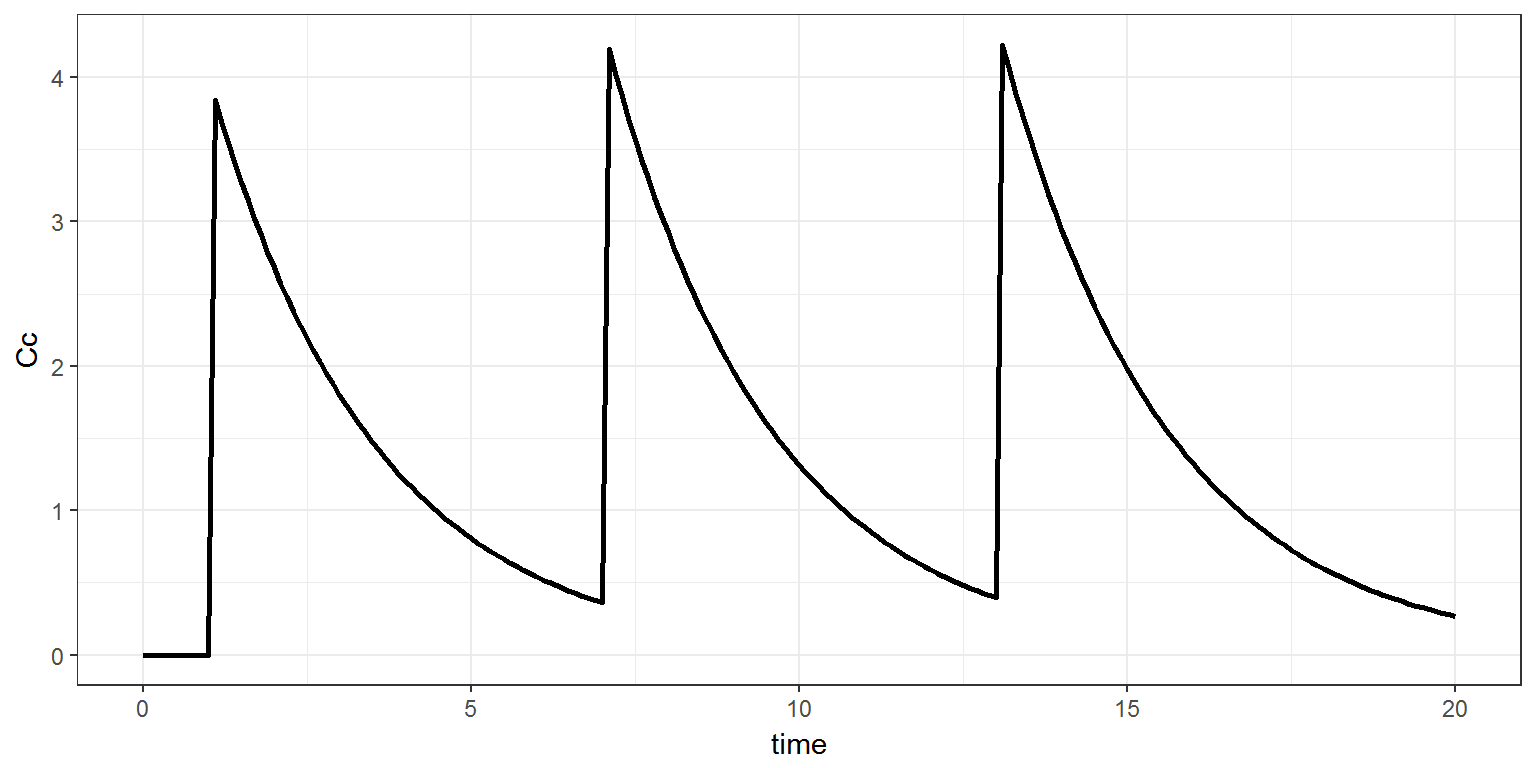Different amounts can be used by defining adm$amount as a vector.

adm <- list(time=c(1, 7, 13), amount=c(40, 20, 10))
res <- simulx(model='model/pk1a.txt', parameter=p, output=Cc, treatment=adm)
print(ggplot(data=res$Cc, aes(x=time, y=Cc)) + geom_line(size=1))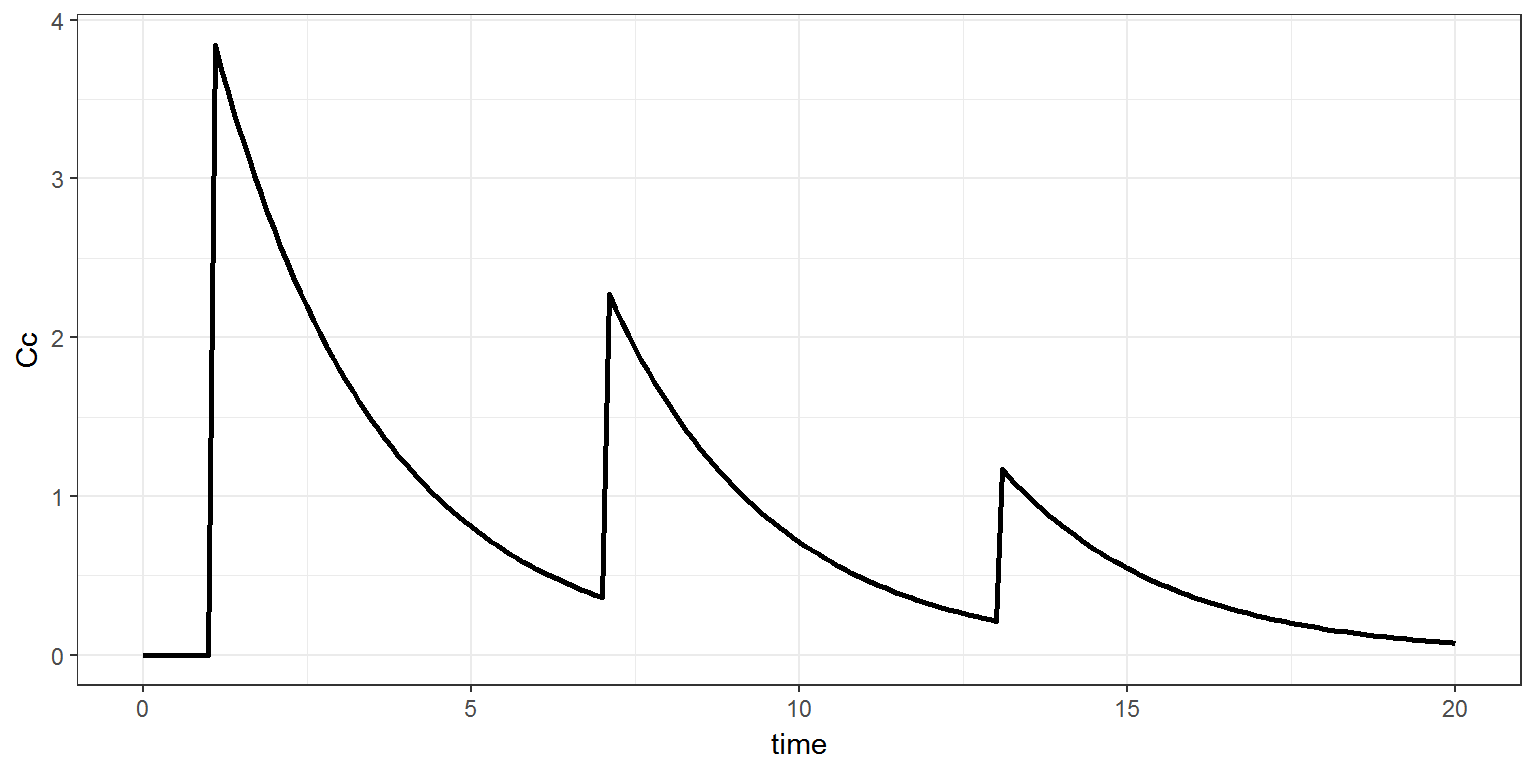## 2.5 Infusion administration An infusion is defined by adding the field rate (infusion rate) to the list treatment. adm <- list(time=c(1, 7, 13), amount=c(40, 20, 10), rate=8) res <- simulx(model='model/pk1a.txt', parameter=p, output=Cc, treatment=adm) print(ggplot(data=res$Cc, aes(x=time, y=Cc)) + geom_line(size=1))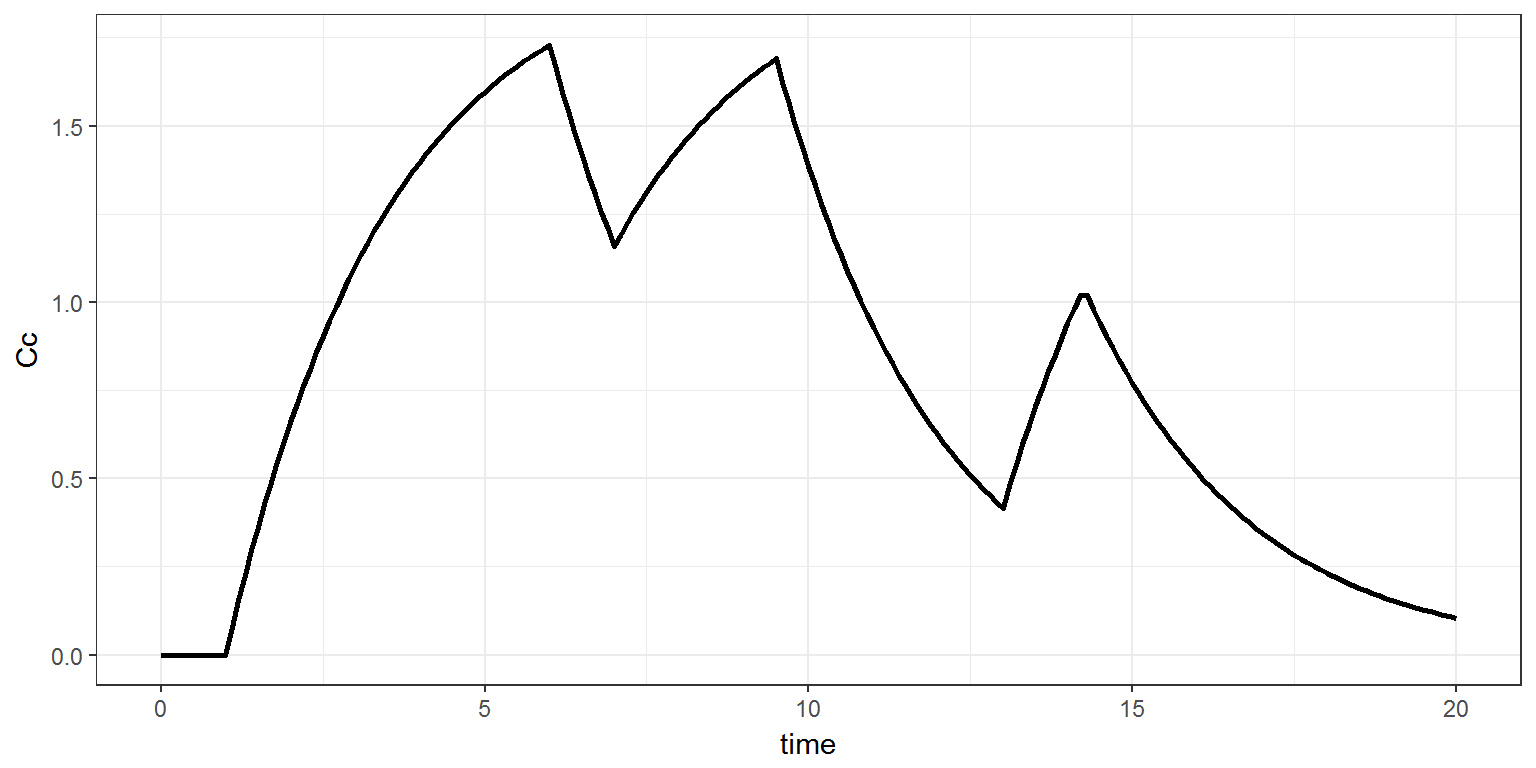Different rates can be used by defining rate as a vector.

adm <- list(time=c(1, 7, 13), amount=c(40, 20, 10), rate=c(8, 5, 4))
res <- simulx(model='model/pk1a.txt', parameter=p, output=Cc, treatment=adm)
print(ggplot(data=res$Cc, aes(x=time, y=Cc)) + geom_line(size=1))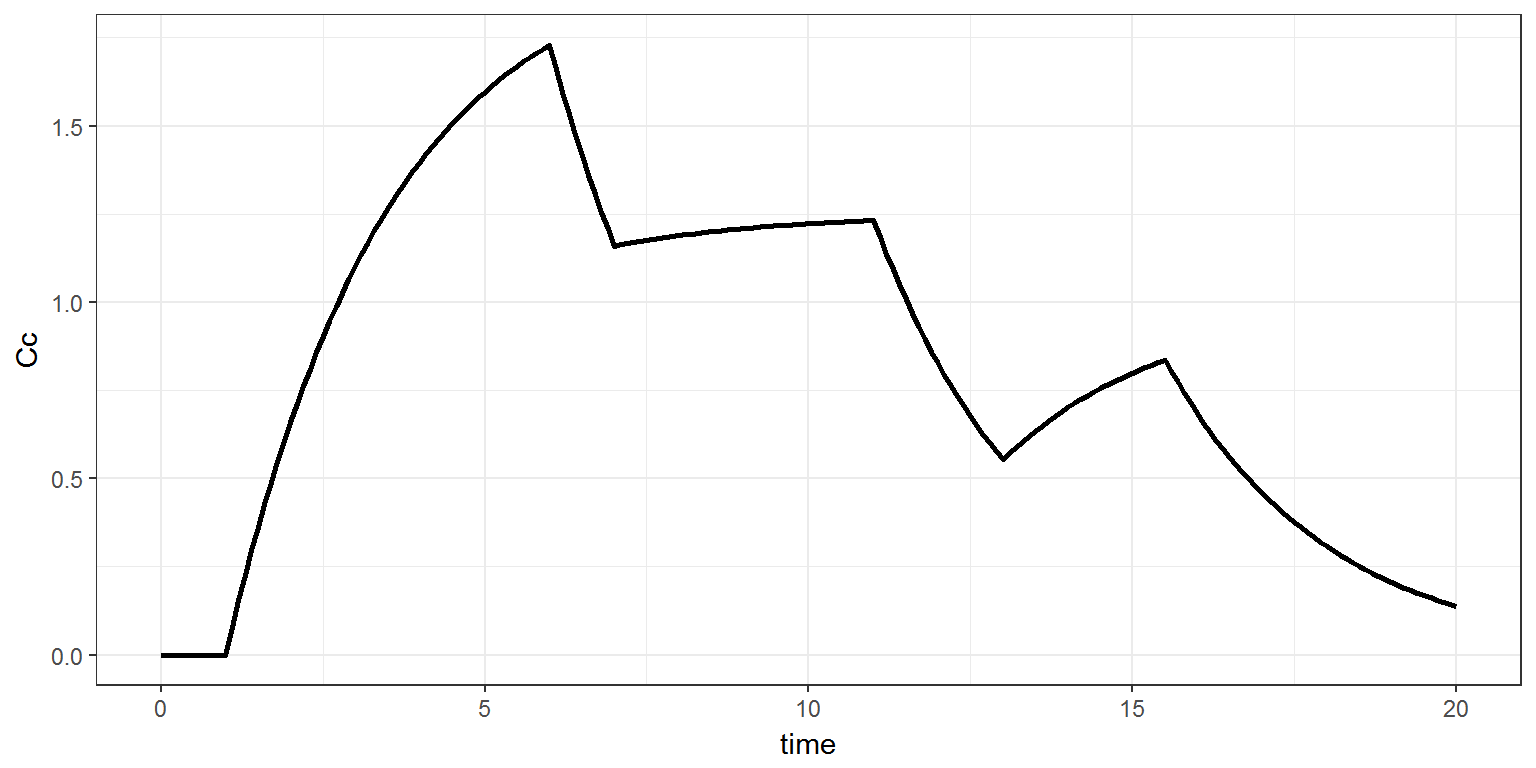Instead of the infusion rate, the duration of the infusion can be equivalently defined as tinf, with tinf=amount/rate adm <- list(time=c(1, 7, 13), amount=c(40, 20, 10), tinf=c(5, 4, 2.5)) res <- simulx(model='model/pk1a.txt', parameter=p, output=Cc, treatment=adm) print(ggplot(data=res$Cc, aes(x=time, y=Cc)) + geom_line(size=1))## 2.6 Defining several dosage regimens

It is also possible to define several dosage regimens using the input argument group. In this example, each element of group has its own dosage regimen

adm1 <- list(time=seq(0,20, by=6), amount=40, tinf=2)
adm2 <- list(time=seq(0,20, by=3), amount=20, tinf=1)
print(ggplot(data=res$Cc, aes(x=time, y=Cc, colour=id)) + geom_line(size=1))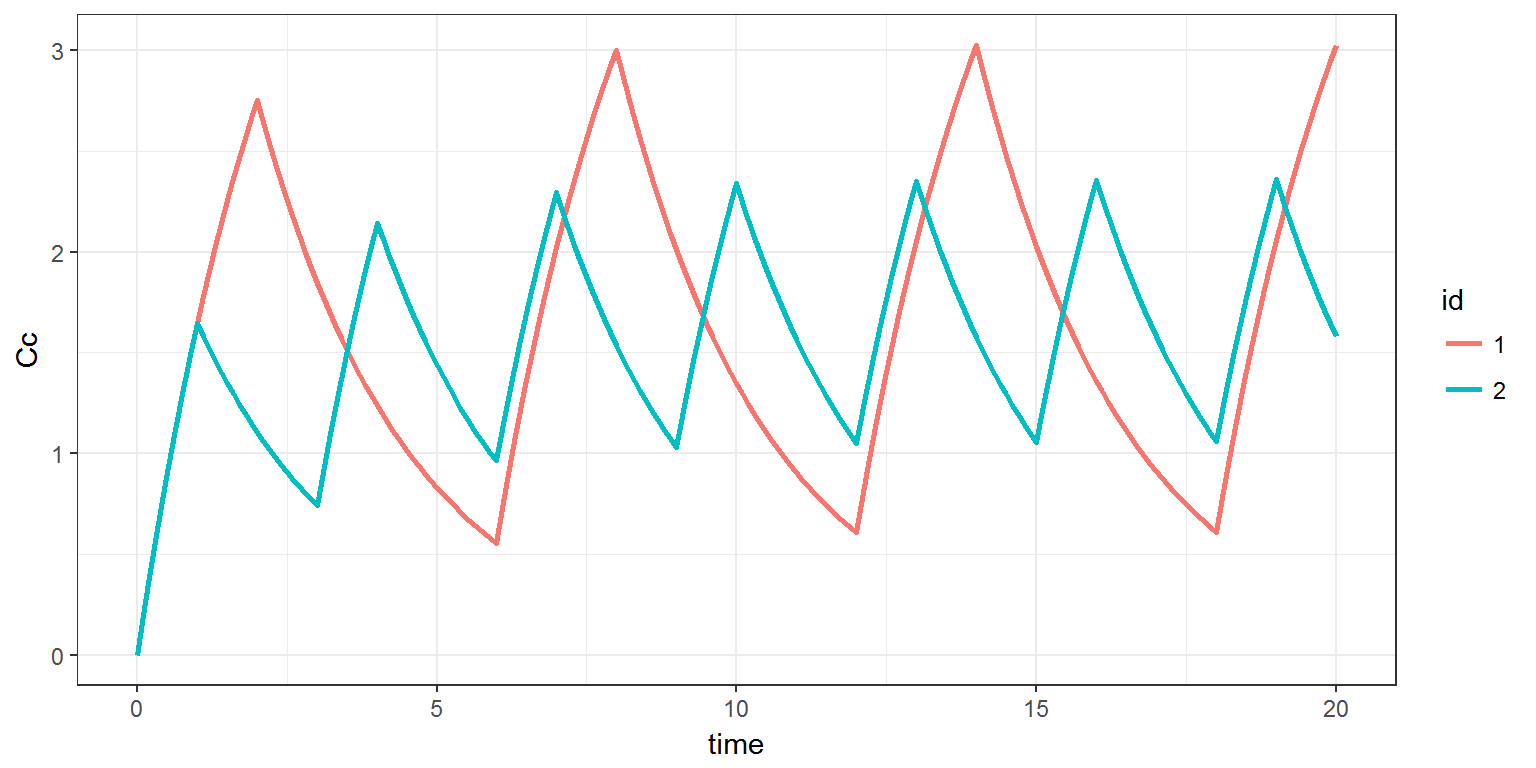## 2.7 Defining the target compartment Instead of defining the target compartment in the PK block of the Mlxtran code, it is possible to define it in the R code. Here, the Mlxtran pk1b.txt code has no block PK: [LONGITUDINAL] input = {V, Cl} EQUATION: k = Cl/V ddt_Ac = -k*Ac Cc=Ac/V  The target compartement (i.e. the central compartment which amount is $$A_c$$) is defined as an additional field target of treatment. adm <- list(target="Ac", time=3, amount=40) res <- simulx(model='model/pk1b.txt', parameter=p, output=Cc, treatment=adm) print(ggplot(data=res$Cc, aes(x=time, y=Cc)) + geom_line(size=1))